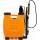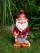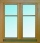# Contract

8 employees complete the contract for 65 hours. After 17 hours, 3 employees had to leave for another job. How many more hours will the job on contract be fulfilled?

Result

t =  76.8 h

#### Solution:

17*8+t*(8-3)=65*8

5t = 384

t = 3845 = 76.8

Calculated by our simple equation calculator.

Leave us a comment of example and its solution (i.e. if it is still somewhat unclear...):

Showing 0 comments:Be the first to comment!#### To solve this example are needed these knowledge from mathematics:

Do you have a linear equation or system of equations and looking for its solution? Or do you have quadratic equation?

## Next similar examples:

1. Working together on projectTwo employees working on the project. First make it alone for 20 days the second for 24 days. How many days will take to complete project if 5 days they work together then first will take two days holiday and after returning they completes a project toget
2. WorkersTwo workers will construct the stage at the festival in 54 hours. Organizers need to shorten the assembly time by 42 hours. How many workers must the assembly company send?
3. NovakNovak needed to dig up three of the same pit in the garden. The first pit dug father alone for 15 hours. His second dig son helped him and it did that in six hours. The third pit dug son himself. How long it took him?
4. SprayersThe vineyard can be sprayed first sprayer in 5 hours and with the second in 8 hours. How long would it take if we use sprayers both at once?
5. DwarfsFour dwarfs would prepare firewood for Snow White in580 minutes. After an hour and a half they recruit friends so that finished preparing the wood for 280 minutes. How many dwarfs they recruited?
6. Construction of roadFor the construction of the road dam, it is supposed to transport six trucks a total of 18 days. After three days, two more cars were sent to the construction site. How long will it take to carry the material?
7. HighwaySix trucks will be used to build the roadside 18 days. After 3 days, two more trucks were sent to build. How long will it take for material move?
8. Workers 9If two workers fix two windows in an hour how many windows can 18 workers fix in the same two windows?
9. EquationSolve the equation: 1/2-2/8 = 1/10; Write the result as a decimal number.
10. Six yearsIn six years Jan will be twice as old as he was six years ago. How old is he?
11. Unknown number 24f we add 20, we get 50% of its triple. What is this unknown number?
12. Unknown numberIdentify unknown number which 1/5 is 40 greater than one tenth of that number.
13. 1.5 divided1.5 divided by 1 = w divided by 4
14. Hectoliters of waterThe pool has a total of 126 hectoliters of water. The first pump draws 2.1 liters of water per second. A second pump pumps 3.5 liters of water per second. How long will it take both pumps to drain four-fifths of the water at the same time?
15. Greg and BillGreg is 18 years old. He is 6 less than 4 times Bill's age. How old is Bill?
16. Simple equationSolve for x: 3(x + 2) = x - 18
17. GivenGiven 2x =0.125 find the value of x